GATE  >  Mining Engineering - MN 2016 GATE Paper (Practice Test)

# Mining Engineering - MN 2016 GATE Paper (Practice Test) - GATE

Test Description

## 65 Questions MCQ Test GATE Past Year Papers for Practice (All Branches) - Mining Engineering - MN 2016 GATE Paper (Practice Test)

Mining Engineering - MN 2016 GATE Paper (Practice Test) for GATE 2023 is part of GATE Past Year Papers for Practice (All Branches) preparation. The Mining Engineering - MN 2016 GATE Paper (Practice Test) questions and answers have been prepared according to the GATE exam syllabus.The Mining Engineering - MN 2016 GATE Paper (Practice Test) MCQs are made for GATE 2023 Exam. Find important definitions, questions, notes, meanings, examples, exercises, MCQs and online tests for Mining Engineering - MN 2016 GATE Paper (Practice Test) below.
Solutions of Mining Engineering - MN 2016 GATE Paper (Practice Test) questions in English are available as part of our GATE Past Year Papers for Practice (All Branches) for GATE & Mining Engineering - MN 2016 GATE Paper (Practice Test) solutions in Hindi for GATE Past Year Papers for Practice (All Branches) course. Download more important topics, notes, lectures and mock test series for GATE Exam by signing up for free. Attempt Mining Engineering - MN 2016 GATE Paper (Practice Test) | 65 questions in 180 minutes | Mock test for GATE preparation | Free important questions MCQ to study GATE Past Year Papers for Practice (All Branches) for GATE Exam | Download free PDF with solutions
 1 Crore+ students have signed up on EduRev. Have you?
Mining Engineering - MN 2016 GATE Paper (Practice Test) - Question 1

### Q. 1 – Q. 5 carry one mark each. Q. The volume of a sphere of diameter 1 unit is ________ than the volume of a cube of side 1 unit.

Mining Engineering - MN 2016 GATE Paper (Practice Test) - Question 2

### The unruly crowd demanded that the accused be _____________ without trial.

Mining Engineering - MN 2016 GATE Paper (Practice Test) - Question 3

### Choose the statement(s) where the underlined word is used correctly: (i) A "prone" is a dried plum. (ii) He was lying "prone" on the floor. (iii) People who eat a lot of fat are "prone" to heart disease.

Mining Engineering - MN 2016 GATE Paper (Practice Test) - Question 4

Fact: If it rains, then the field is wet.
(i) It rains
(ii) The field is not wet
(iii) The field is wet
(iv) It did not rain
Which one of the options given below is NOT logically possible, based on the given fact?

Mining Engineering - MN 2016 GATE Paper (Practice Test) - Question 5

The average weight of 16 boys in a class is 50.25 kg and that of the remaining 8 boys is 45.15 kg. Find the average weights of all the boys in the class.

Detailed Solution for Mining Engineering - MN 2016 GATE Paper (Practice Test) - Question 5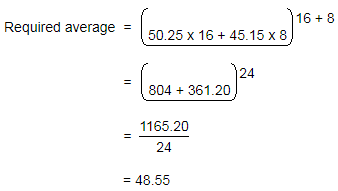Mining Engineering - MN 2016 GATE Paper (Practice Test) - Question 6

Q. 6 – Q. 10 carry two marks each.

Q.

Students taking an exam are divided into two groups, P and Q such that each group has the same
number of students. The performance of each of the students in a test was evaluated out of 200
marks. It was observed that the mean of group P was 105, while that of group Q was 85. The
standard deviation of group P was 25, while that of group Q was 5. Assuming that the marks were
distributed on a normal distribution, which of the following statements will have the highest
probability of being TRUE?

Mining Engineering - MN 2016 GATE Paper (Practice Test) - Question 7

A smart city integrates all modes of transport, uses clean energy and promotes sustainable use of
resources. It also uses technology to ensure safety and security of the city, something which critics
argue, will lead to a surveillance state.
Which of the following can be logically inferred from the above paragraph?

(i) All smart cities encourage the formation of surveillance states.
(ii) Surveillance is an integral part of a smart city.
(iii) Sustainability and surveillance go hand in hand in a smart city.
(iv) There is a perception that smart cities promote surveillance.

Mining Engineering - MN 2016 GATE Paper (Practice Test) - Question 8

Find the missing sequence in the letter series.
B, FH, LNP, _ _ _ _.

Mining Engineering - MN 2016 GATE Paper (Practice Test) - Question 9

The binary operation ? is defined as a ? b = ab+(a+b), where a and b are any two real numbers.
The value of the identity element of this operation, defined as the number x such that a ? x = a, for
any a, is

Mining Engineering - MN 2016 GATE Paper (Practice Test) - Question 10

Which of the following curves represents the function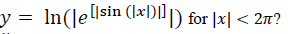Mining Engineering - MN 2016 GATE Paper (Practice Test) - Question 11

Q. 1 – Q. 25 carry one mark each

Q.

The differential of the equation, x2 + y2 =1, with respect to x is

Mining Engineering - MN 2016 GATE Paper (Practice Test) - Question 12

If [A][B] = [I ] then

Mining Engineering - MN 2016 GATE Paper (Practice Test) - Question 13

X4 +C is the general integral of

Mining Engineering - MN 2016 GATE Paper (Practice Test) - Question 14

Sinh (x) is

Mining Engineering - MN 2016 GATE Paper (Practice Test) - Question 15

Identify the correct statement.
NONEL is used for surface connection of the blast holes in order to

Mining Engineering - MN 2016 GATE Paper (Practice Test) - Question 16

Identify the pattern of surface blasting given in the figure. The values of delay time, in ms,
are given against each blasthole.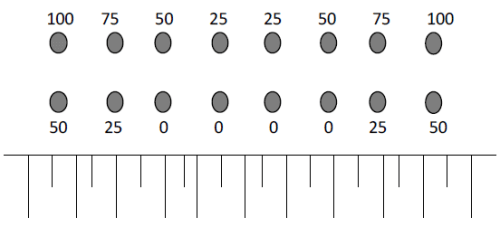Mining Engineering - MN 2016 GATE Paper (Practice Test) - Question 17

Identify the initiation sequence which is NOT possible for surface blasting.

Mining Engineering - MN 2016 GATE Paper (Practice Test) - Question 18

Parallel holes at right angles to the face with some holes uncharged are associated with the following shot hole pattern

Mining Engineering - MN 2016 GATE Paper (Practice Test) - Question 19

Bieniawski’s Rock Mass Rating considers the parameters: RQD, spacing of joints, condition of
joints, ground water condition, and

Mining Engineering - MN 2016 GATE Paper (Practice Test) - Question 20

The unruly crowd demanded that the accused be without trial.

Mining Engineering - MN 2016 GATE Paper (Practice Test) - Question 21

Identify the uniaxial compressive loading condition from the following four Mohr circles.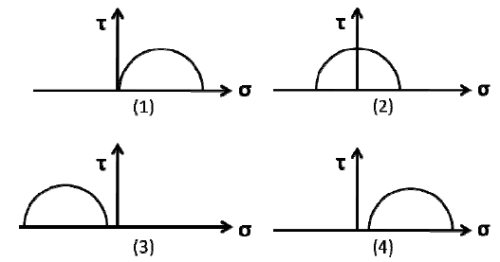Mining Engineering - MN 2016 GATE Paper (Practice Test) - Question 22

Out of the given stress-strain curves, identify the rock type that is most prone to rock burst.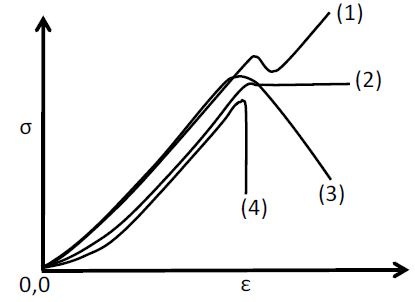*Answer can only contain numeric values
Mining Engineering - MN 2016 GATE Paper (Practice Test) - Question 23

A longwall panel of width 120 m is extracted at a depth of 200 m. Critical subsidence is reached
when the panel length becomes 150 m. If the seam were to be worked at a depth of 300 m, critical
subsidence would be observed at a panel length, in m, of _______________

Important : you should answer only the numeric value

Mining Engineering - MN 2016 GATE Paper (Practice Test) - Question 24

The support system followed along the goaf edge in a depillaring panel is

Mining Engineering - MN 2016 GATE Paper (Practice Test) - Question 25

Which one of the following ropes CANNOT be an effective cable bolt?

Mining Engineering - MN 2016 GATE Paper (Practice Test) - Question 26

In metalliferous mines, the sublevel interval does NOT depend on

Mining Engineering - MN 2016 GATE Paper (Practice Test) - Question 27

Jack hammer does NOT contain

Mining Engineering - MN 2016 GATE Paper (Practice Test) - Question 28

At the inlet of a mine roadway, the dry and wet bulb temperatures of air are 380Cand 290C,
respectively. At the outlet, the corresponding temperatures are 320C and 290C, respectively. The
process of heat transfer in the airway is described as

Mining Engineering - MN 2016 GATE Paper (Practice Test) - Question 29

Underground coal mines are in principle ventilated by exhausting system, so that

Mining Engineering - MN 2016 GATE Paper (Practice Test) - Question 30

Identify the WRONG statement.
Pit bottom air lock

Mining Engineering - MN 2016 GATE Paper (Practice Test) - Question 31

Identify the WRONG statement.
The ‘temperature inversion’ of the atmosphere in surface mines aggravates the problem of

Mining Engineering - MN 2016 GATE Paper (Practice Test) - Question 32

In a CO self rescuer, the purpose of the calcium bromide and lithium chloride mixture is to

Mining Engineering - MN 2016 GATE Paper (Practice Test) - Question 33

IRR of a project is the discount rate at which

Mining Engineering - MN 2016 GATE Paper (Practice Test) - Question 34

For the critical path network shown, the slack for the activity ‘b’, in months, is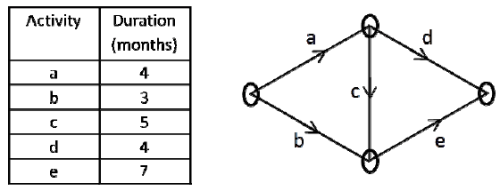Mining Engineering - MN 2016 GATE Paper (Practice Test) - Question 35

The three axes comprising the numerical codification of resources, as per the UNFC, are

*Answer can only contain numeric values
Mining Engineering - MN 2016 GATE Paper (Practice Test) - Question 36

Q. 36 – Q. 65 carry two marks each

Q.

Equations of two planes are z = 4 and z = 4 + 3x . The included angle between the two planes in
degrees, is ____________

*Answer can only contain numeric values
Mining Engineering - MN 2016 GATE Paper (Practice Test) - Question 37

A force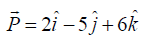acts on a particle. The particle is moved from point A to point B, where
the position vectors of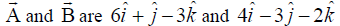respectively. The work done is
____________

(Important : you should answer only the numeric value)

*Answer can only contain numeric values
Mining Engineering - MN 2016 GATE Paper (Practice Test) - Question 38

The value of x in the simultaneous equations is ______________

3x+y+2z=3

2x-3y-z=-3

x+2y+z=4

(Important : you should answer only the numeric value)

*Answer can only contain numeric values
Mining Engineering - MN 2016 GATE Paper (Practice Test) - Question 39

Two persons P and Q toss an unbiased coin alternately on an understanding that whoever gets the
head first wins. If P starts the game, then the probability of P winning the game is ____________

*Answer can only contain numeric values
Mining Engineering - MN 2016 GATE Paper (Practice Test) - Question 40

Data pertaining to a surface bench blast is given below: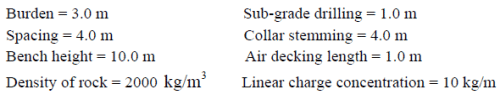The powder factor of the blast, in kg/tonne, is _______

Mining Engineering - MN 2016 GATE Paper (Practice Test) - Question 41

Match the following for a typical slurry explosive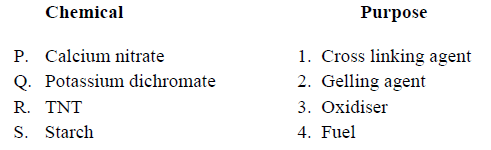*Answer can only contain numeric values
Mining Engineering - MN 2016 GATE Paper (Practice Test) - Question 42

A 10 m thick coal block is excavated by a contractor at a cost of Rs. 40 per m3 . The excavated
area, measured in the mine plan, is found to be50 cm2 . If the mine plan has been drawn to a scale
of 1:1000, the payment to be made to the contractor, in lakhs of Rs., is _______

(Important : you should answer only the numeric value)

*Answer can only contain numeric values
Mining Engineering - MN 2016 GATE Paper (Practice Test) - Question 43

Two vertical shafts of a mine have the following parameters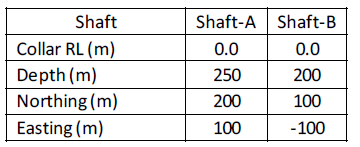The gradient of the drift connecting the shaft bottoms, in degrees, is_____

Mining Engineering - MN 2016 GATE Paper (Practice Test) - Question 44

For a station ‘A’ on the Earth’s surface, as shown in the figure, match the following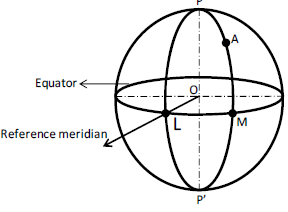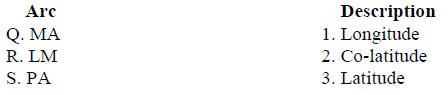Mining Engineering - MN 2016 GATE Paper (Practice Test) - Question 45

Match the following for the prismatic compass shown below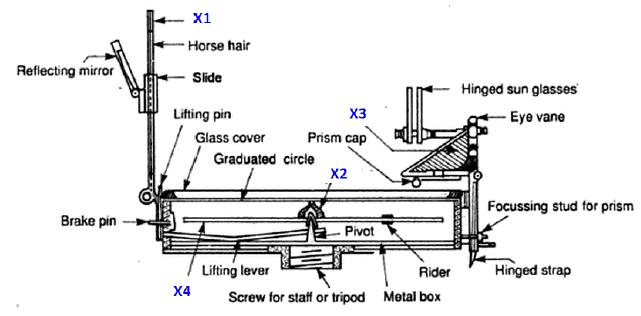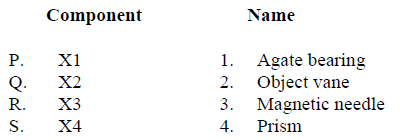*Answer can only contain numeric values
Mining Engineering - MN 2016 GATE Paper (Practice Test) - Question 46

A ladder placed against a frictionless wall at an inclination of 60with horizontal, is in a state of
limiting equilibrium. The ladder has a length of 13 m and a uniform mass of 4 kg/m. The
coefficient of friction between the ladder and the floor is ______

*Answer can only contain numeric values
Mining Engineering - MN 2016 GATE Paper (Practice Test) - Question 47

A cubical rock sample is enclosed between two fixed hard steel plates as shown in the figure below.
The modulus of elasticity and Poisson’s ratio of the rock are 2 GPa and 0.25, respectively. If the
rock is subjected to the stresses as shown in the figure, the strain in x-direction, in mm/m, is
_______.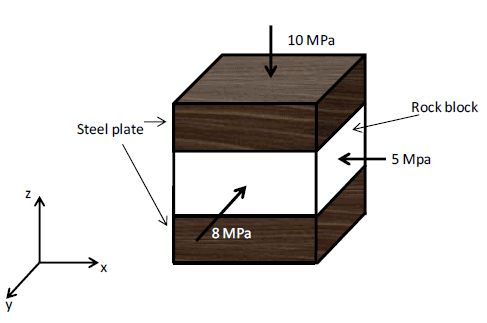*Answer can only contain numeric values
Mining Engineering - MN 2016 GATE Paper (Practice Test) - Question 48

In a hydrostatic stress field, point A is in the middle of two circular openings as shown in the
figure. The radial stress, in MPa, at point A is _________.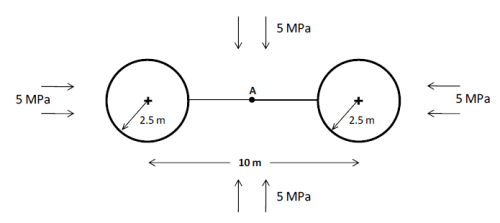Mining Engineering - MN 2016 GATE Paper (Practice Test) - Question 49

Curves (a) and (b) represent the stress distributions along the length of a ‘full column grouted bolt’
shown in the figure. Curves (a) and (b) are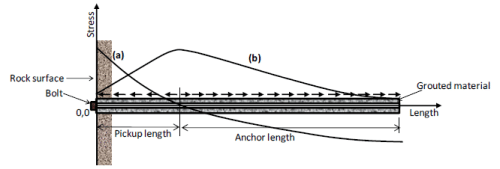Mining Engineering - MN 2016 GATE Paper (Practice Test) - Question 50

Match the following mechanical properties with the formulae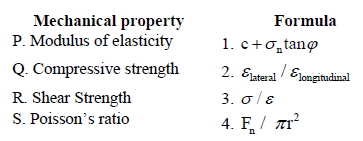*Answer can only contain numeric values
Mining Engineering - MN 2016 GATE Paper (Practice Test) - Question 51

A skip of 10 tonne capacity hoists ore through a 1000 m deep shaft at a speed of 20 m/s. The skip
and 1.5 min, respectively. The maximum hourly capacity of the hoisting system, in tonnes, is
_____________

Mining Engineering - MN 2016 GATE Paper (Practice Test) - Question 52

Match the following: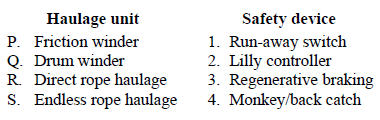*Answer can only contain numeric values
Mining Engineering - MN 2016 GATE Paper (Practice Test) - Question 53

In the gear assembly shown, the rpm of Gear 1 is 600. The number of teeth in Gear 1, Gear 2, Gear
3, Gear 4, Gear 5 and Gear 6 is 30, 45, 15, 20, 10 and 30, respectively. The rpm of Gear 6 is
______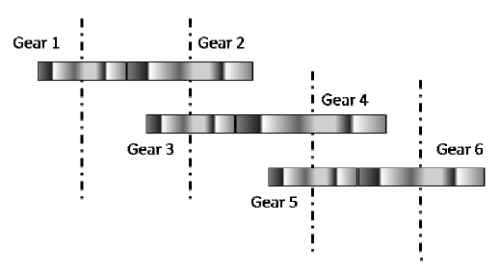(Important : you should answer only the numeric value)

*Answer can only contain numeric values
Mining Engineering - MN 2016 GATE Paper (Practice Test) - Question 54

An operating surface mine is proposed to be deepened by 30 m as shown in the figure. If the
density of the ore is 2.4 tonne m3 , the incremental stripping ratio for the deepening, in
m3 tonne , is ______.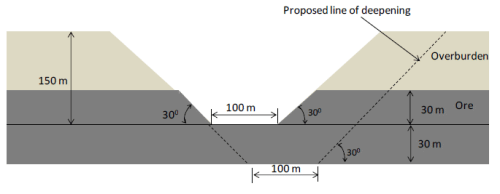*Answer can only contain numeric values
Mining Engineering - MN 2016 GATE Paper (Practice Test) - Question 55

From an openpit sump, mine water is lifted using a 250 m long straight pipeline laid along a
gradient of 340 . The pumping rate is 500 gpm (1 gallon = 3.8 litres). Additional head loss due to
pipe friction can be considered to be 10% of head lifted. At an overall efficiency of 70%, the
electric power consumed by the pump, in kW, is __________.

Mining Engineering - MN 2016 GATE Paper (Practice Test) - Question 56

With reference to Coward diagram, match the following in the context of explosibility of a mixture
of ‘normal air’ and ‘methane’.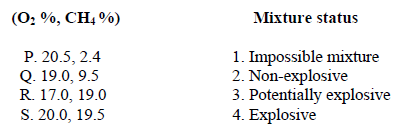*Answer can only contain numeric values
Mining Engineering - MN 2016 GATE Paper (Practice Test) - Question 57

A U-tube manometer is subjected to differential pressure as shown. If specific gravity of kerosene
is 0.8, the value of (P1 - P2 ), in Pa, is_________.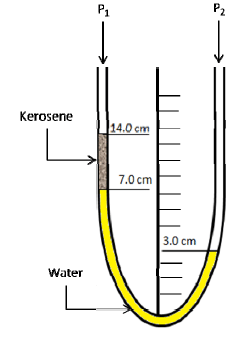*Answer can only contain numeric values
Mining Engineering - MN 2016 GATE Paper (Practice Test) - Question 58

An air stream having an enthalpy of 100 kJ/kgda, is flowing at 20 kgda/s. It is cooled by water at
temperature 100C circulating in a cooling coil at a flow rate of 10.0 l/s. If the return temperature of
water is 200C , the enthalpy of the cooled air, in kJ/kgda, is ___________.
(Specific heat of water: 4.18 kJ kg 0C ; kgda: kg of dry air).

*Answer can only contain numeric values
Mining Engineering - MN 2016 GATE Paper (Practice Test) - Question 59

The static pressure characteristic of a mine fan is as shown. If the mine resistance is 0.3 Ns2/ m8 ,
the quantity generated by the fan, in m3/s , is _______.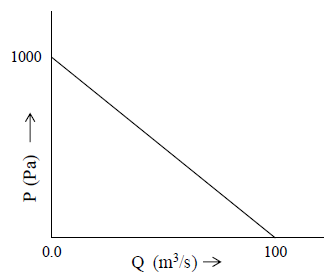Mining Engineering - MN 2016 GATE Paper (Practice Test) - Question 60

In the context of ventilation plan symbols, match the following: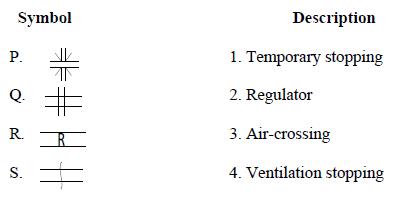*Answer can only contain numeric values
Mining Engineering - MN 2016 GATE Paper (Practice Test) - Question 61

A mill concentrate, having 25% copper, is proposed to be sold at Rs. 1,25,000 per tonne. The grade
of the deposit is 0.8% Cu and the overall cost of mining and milling is Rs. 2,520 per tonne of ore.
At a recovery of 75%, the estimated profit, in Rs./tonne of concentrate, is __________

*Answer can only contain numeric values
Mining Engineering - MN 2016 GATE Paper (Practice Test) - Question 62

Copper grade distribution in an ore body has the probability density function, f (x) , as shown in
the figure. The average grade of the deposit, in % Cu, is ______.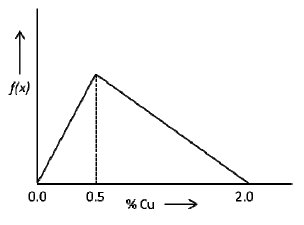*Answer can only contain numeric values
Mining Engineering - MN 2016 GATE Paper (Practice Test) - Question 63

The semivariogram shown belongs to a bauxite deposit. The expected difference in the Al2O3 (%)
values between two boreholes separated by a distance of 200 m is _______.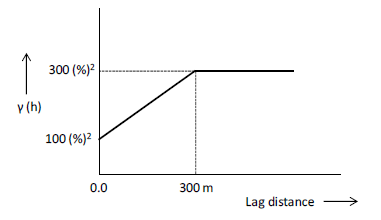(Important : you should answer only the numeric value)

Mining Engineering - MN 2016 GATE Paper (Practice Test) - Question 64

A surface mine has 15 identical dumpers and two shovels. For shovel 1, the dumper cycle time is
30 min and the shovel loading time is 5 min. For shovel 2, the dumper cycle time is 32 min and the
shovel loading time is 4.0 min. Based on match factor optimisation (equitable match factor), the
ideal allocation of dumpers to shovel 1 and shovel 2, respectively is

*Answer can only contain numeric values
Mining Engineering - MN 2016 GATE Paper (Practice Test) - Question 65

The composited grade value, in %, between the RLs 10 m to 20 m for the following borehole
configuration is ________________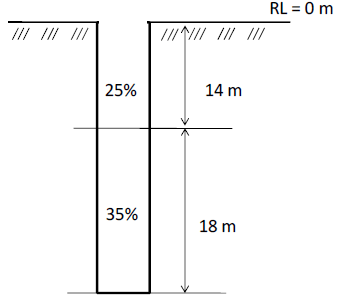(Important : you should answer only the numeric value)

## GATE Past Year Papers for Practice (All Branches)

407 docs|127 tests
Information about Mining Engineering - MN 2016 GATE Paper (Practice Test) Page
In this test you can find the Exam questions for Mining Engineering - MN 2016 GATE Paper (Practice Test) solved & explained in the simplest way possible. Besides giving Questions and answers for Mining Engineering - MN 2016 GATE Paper (Practice Test), EduRev gives you an ample number of Online tests for practice

## GATE Past Year Papers for Practice (All Branches)

407 docs|127 tests(Scan QR code)